Question

Find the volume of the parallelepiped spanned by the vectors u=〈3,−2,2〉, v=〈1,0,1〉, and w=〈−2,1,−5〉. Write the...

Find the volume of the parallelepiped spanned by the vectors u=〈3,−2,2〉, v=〈1,0,1〉, and w=〈−2,1,−5〉. Write the exact answer. Do not round.

We need at least 10 more requests to produce the answer.

0 / 10 have requested this problem solution

The more requests, the faster the answer.

All students who have requested the answer will be notified once they are available.

Earn Coins

Coins can be redeemed for fabulous gifts.

Similar Homework Help Questions
• volume of the parallelepiped build on three vectors

Find the volume of the parallelepiped build on three vectors: u=(1,1,2), v=(3,2,1), w=(1,6,6)

• Vectors : If u and v are vectors such that..

|u|=2 , |v| = 3 and the angle between u and v is pi/3a)Find the exact value for |u - 2v|b) let u = <4,-8,1> , v = <2,1,-2> and w = <3,-4,12> determine if the vectors u,v and w are coplanar. Justify your answerc) Let u,v and w be vectors such that u . (v x w) = 3 Find(i) u.(w x v) (ii) (u x w).v (iii) v.(w x w)

• answer this . will rate after Given the vectors u = (1, -2,1), v= {5,–4,0) and...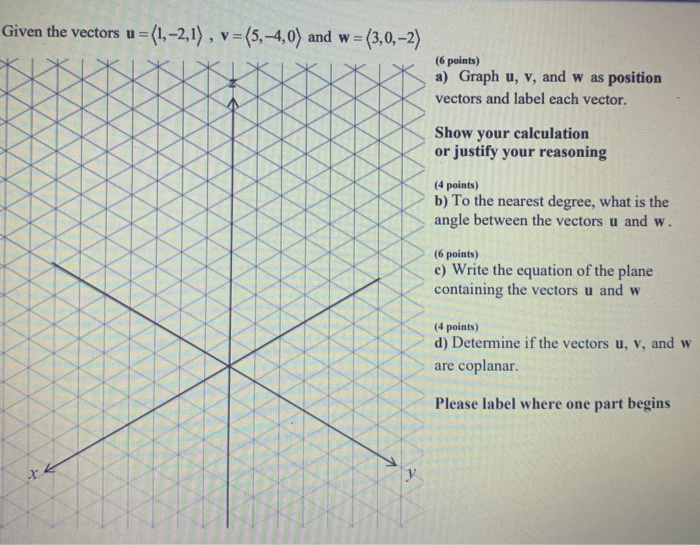answer this . will rate after Given the vectors u = (1, -2,1), v= {5,–4,0) and w = (3,0,-2) (6 points) a) Graph u, v, and was position vectors and label each vector. Show your calculation or justify your reasoning (4 points) b) To the nearest degree, what is the angle between the vectors u and w. (6 points) c) Write the equation of the plane containing the vectors u and w (4 points) d) Determine if the vectors u,...

• b. Find the volume of the parallelepiped spanned by the vectors (t, 0,0), (1,2,-4), (0, t,-1). For what values of t will there be a zero volume? What can you say about the three vectors when the volu...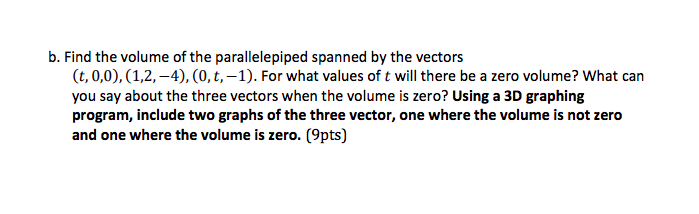b. Find the volume of the parallelepiped spanned by the vectors (t, 0,0), (1,2,-4), (0, t,-1). For what values of t will there be a zero volume? What can you say about the three vectors when the volume is zero? Using a 3D graphing program, include two graphs of the three vector, one where the volume is not zero and one where the volume is zero. (9pts) b. Find the volume of the parallelepiped spanned by the vectors (t, 0,0),...

• 1. Let u - (1,1,2), v = (1,2,1), and w (2,1,1) in R. and consider •...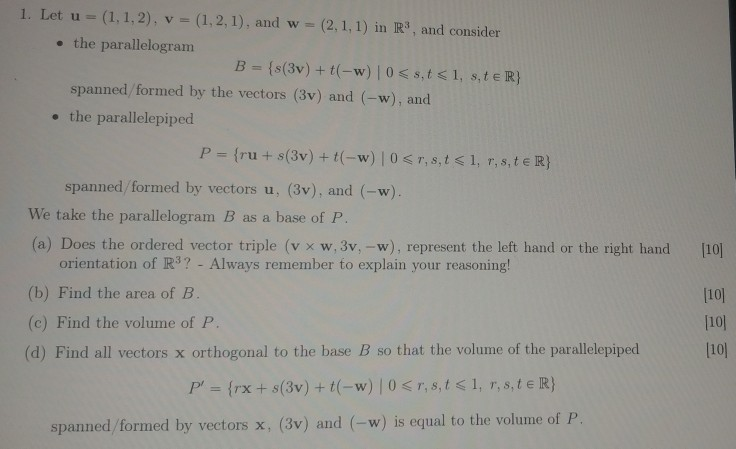1. Let u - (1,1,2), v = (1,2,1), and w (2,1,1) in R. and consider • the parallelogram B = {s(3v) + t-w) 0 <s,t<1, s,te R} spanned/formed by the vectors (3v) and (-w), and • the parallelepiped P = {ru + s(3v) + (-w) 0 <T,,t<1, r, s, t€ R}  spanned formed by vectors u. (3v). and (-w) We take the parallelogram B as a base of P. (a) Does the ordered vector triple (v xw, 3v, -w),...

• Q6. Let W be the subspace of R' spanned by the vectors u. = 3(1, -1,1,1),...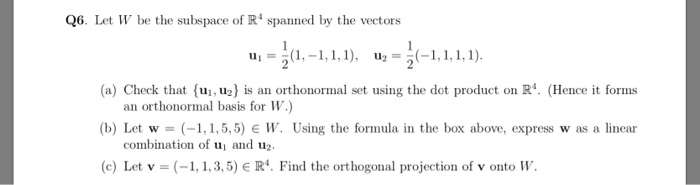Q6. Let W be the subspace of R' spanned by the vectors u. = 3(1, -1,1,1), uz = 5(–1,1,1,1). (a) Check that {uj,uz) is an orthonormal set using the dot product on R. (Hence it forms an orthonormal basis for W.) (b) Let w = (-1,1,5,5) EW. Using the formula in the box above, express was a linear combination of u and u. (c) Let v = (-1,1,3,5) = R'. Find the orthogonal projection of v onto W.

• Problem l: Let u, v and w be three vectors in R3 (a) Prove that wlv +lvlw bisects the angle betwe...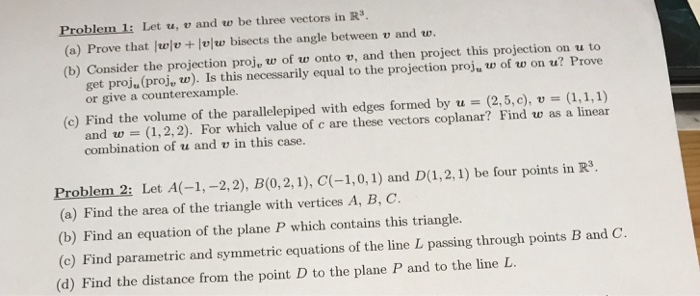Problem l: Let u, v and w be three vectors in R3 (a) Prove that wlv +lvlw bisects the angle between v and w. (b) Consider the projection proj, w of w onto v, and then project this projection on u to get proju (proj, w). Is this necessarily equal to the projection proj, w of w on u? Prove or give a counterexample. (c) Find the volume of the parallelepiped with edges formed by u-(2,5,c), v (1,1,1) and w...

• (3 points) Let W be the subspace of R spanned by the vectors 1and 5 Find the matrix A of the orthogonal projection onto W A- (3 points) Let W be the subspace of R spanned by the vectors 1and 5 F...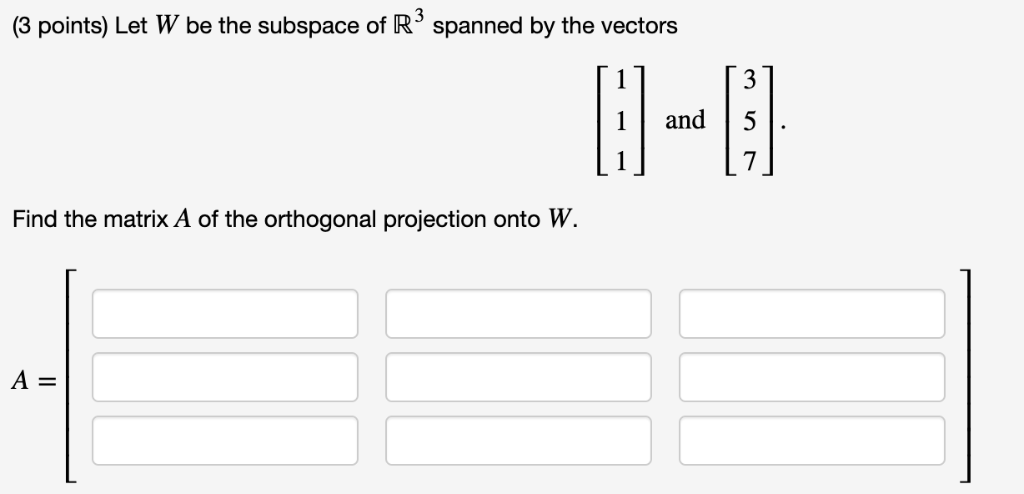(3 points) Let W be the subspace of R spanned by the vectors 1and 5 Find the matrix A of the orthogonal projection onto W A- (3 points) Let W be the subspace of R spanned by the vectors 1and 5 Find the matrix A of the orthogonal projection onto W A-

• ... Let v = , u = , and let W the subspace of R4 spanned by v and u. Find a basis of W .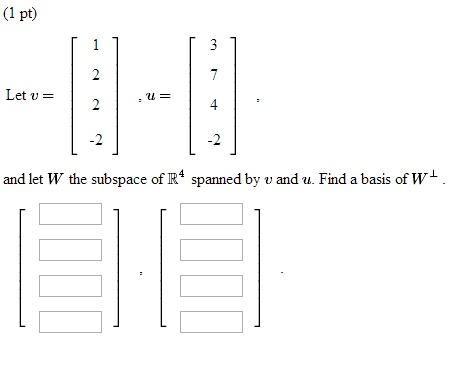... Let v = , u = , and let W the subspace of R4 spanned by v and u. Find a basis of W .

• If "u = (2,2,-1)", "v = (3,-1,0)" and "w = (1,7,8)", verify that "u (dot) (v + w) = u (dot) v + u (dot) w"

If "u = (2,2,-1)", "v = (3,-1,0)" and "w = (1,7,8)", verify that "u (dot) (v + w) = u (dot) v + u (dot) w".My work:LHS:u (dot) (v + w)= (2,2,-1) (dot) (4,6,8)= [(2,2,-1) (dot) (3,-1,0)] + [(2,2,-1) (dot) (1,7,8)]= [u (dot) v] + [u (dot) w]Therefore, LHS = RHSDid I prove this question correctly?